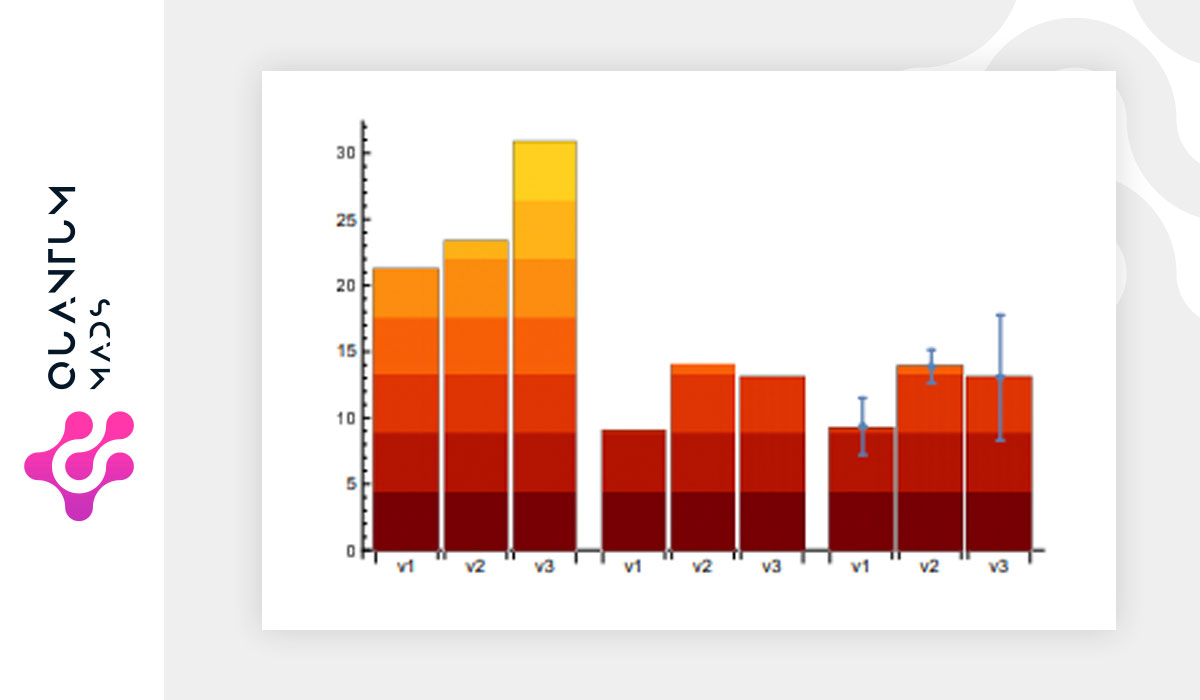Saltear al contenido principalPrediction of financial crashes in a complex financial network is known to be an NP-hard problem, i.e., a problem which cannot be solved efficiently with a classical computer. We experimentally explore a novel approach to this problem by using a D-Wave quantum computer to obtain financial equilibrium more efficiently. To be specific, the equilibrium condition of a nonlinear financial model is embedded into a higher-order unconstrained binary optimization (HUBO) problem, which is then transformed to a spin-1/2 Hamiltonian with at most two-qubit interactions.

The problem is thus equivalent to finding the ground state of an interacting spin Hamiltonian, which can be approximated with a quantum annealer. Our experiment paves the way to study quantitative macroeconomics, enlarging the number of problems that can be handled by current quantum computers.

Read the whole article on arVix

Volver arriba